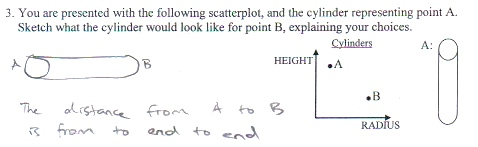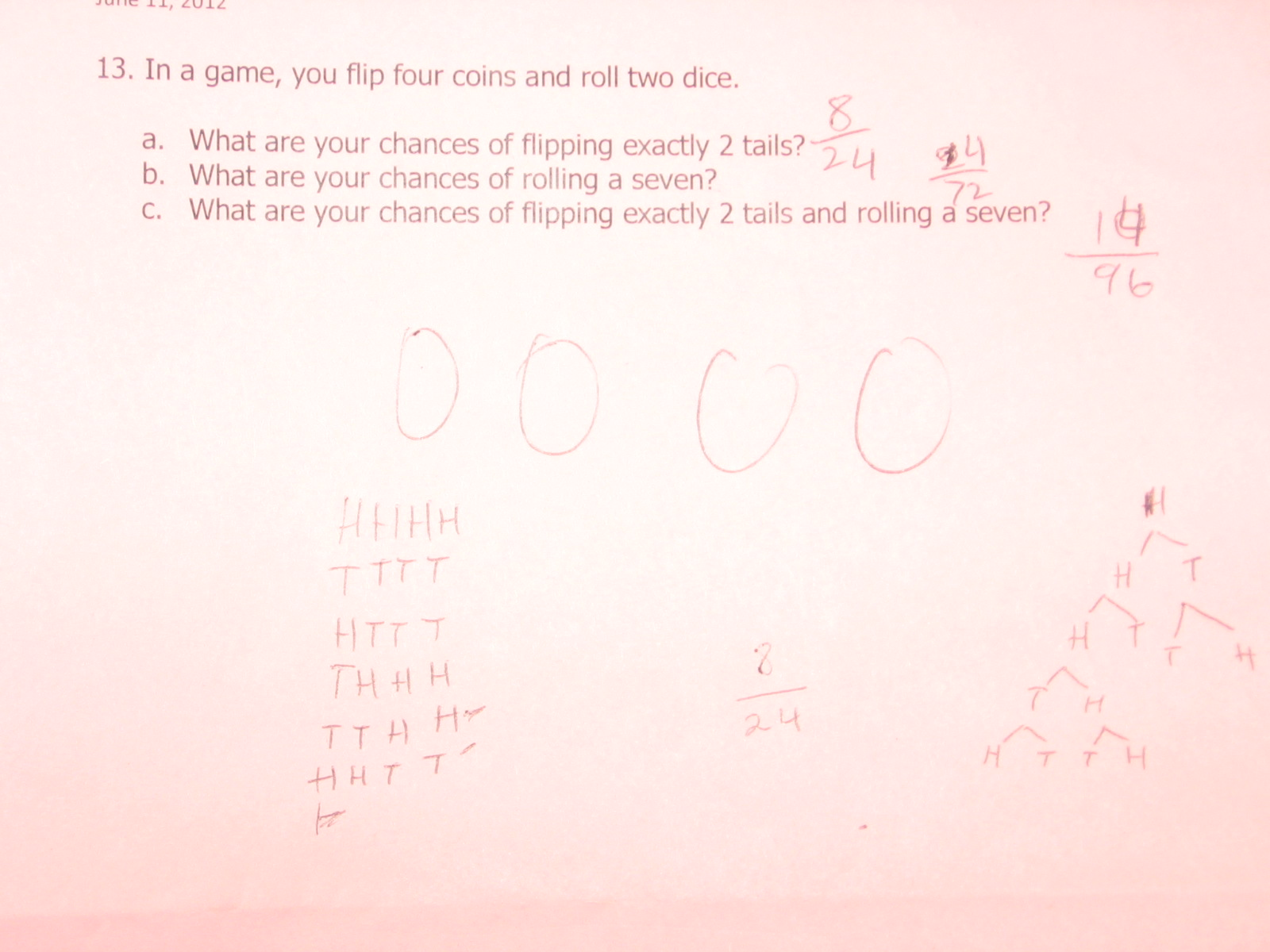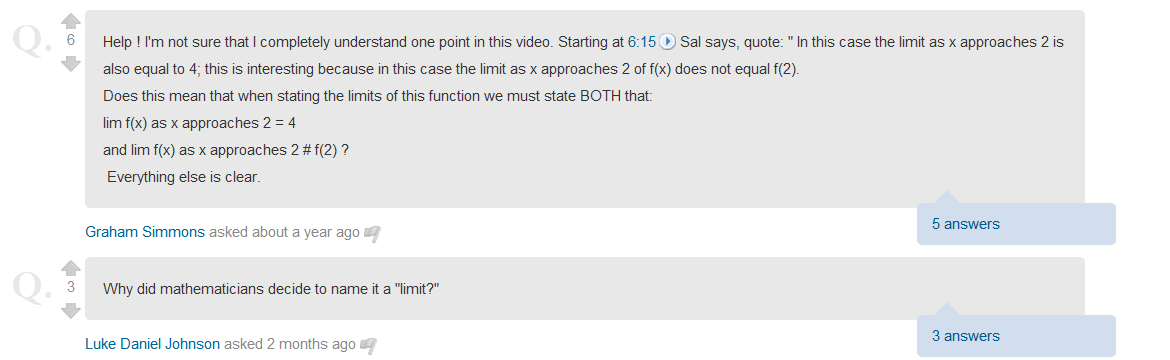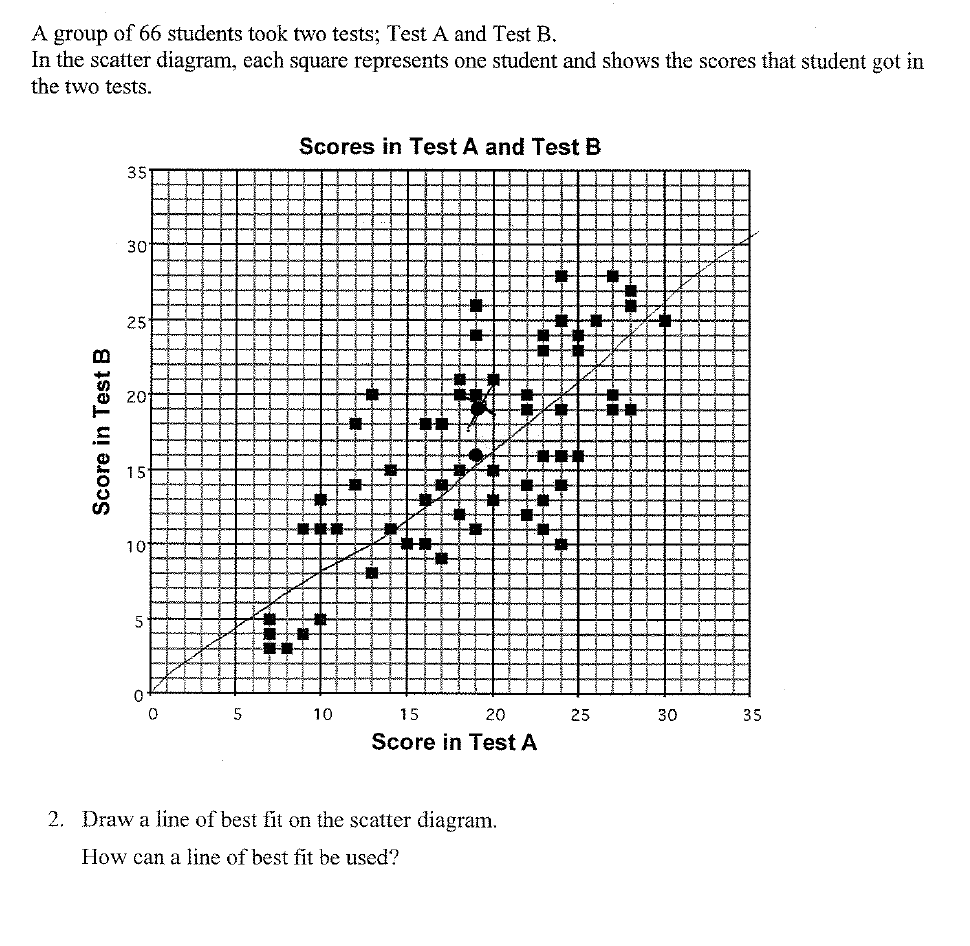Categories

## Cylinder Scatterplot1. This is a really cool question.

2. Gregory Taylor says he doesn’t know what the kid was thinking. Thoughts?

Categories

## What are your chances of flipping exactly 2 tails?Can you explain where each of the student’s responses came from? As in, how did he arrive at 8/24, 14/96, and what is either 24/72 or 14/72.

Categories

## What are your chances of rolling a seven?Where did 12/36 come from in part B?

Categories

## Coin Flipping

I saw this one in class last week:

Probability of flipping 10 heads when flipping 10 coins: (1/2)^10

Probability of flipping 9 heads when flipping 10 coins: (1/2)^9

Categories

## “You ought to get that probability at least once.”

At 1:30, a student says, “if you do that probability 3 times, you ought to get that probability at least once.”

How should I have responded to this student? (I actually have the footage, which we can analyze at some point if we think that’d be fun.)

Categories

## What’s the frequency?Bob Lochel asks: “I am wondering if anyone can conjecture why “3” was featured in both answers, as I can’t wrap my head around it.  Perhaps it was a counting of the number of cells in the row she needed?”

Categories

## Histograms and FrequencyThe submitter reports: “There was some controversy in our department over the fact that the y axis has been labeled as frequency. The y axis should be labeled frequency density. However the majority of students did manage to ignore or relabel the axis correctly. This student has become confused by the whole thing.”

Ignoring the mislabeling of the problem, what evidence do we have about student knowledge?

Thanks to Ian Hopkins for the submission. Follow him on twitter too.

Categories

## Mean (Number of) Girls (and Boys)

“Find the mean number of siblings.”Student 1: (0+1+2+3+4+5)/6 = 2.5
Student 2: (1+4+6+4+2+1)/18=1
Student 3: (1+4+6+4+2+1)/6=3
Student 4: (0+1+2+3+4+5)/18 = 0.83
What sort of misconceptions can you dig out of these student responses? If this were your class, how would you respond?
Categories

I’m sitting on a bunch of Khan Academy questions from users that I marked as very interesting. I never posted them, and I feel a little cheap giving them all their own posts. So I figured I’d just dump the whole lot on you all. These questions reveal interesting things about the way these students are thinking. If you think that you’ve got something interesting to add, either on the diagnosis or prescription side of things, dig into the comments below.

The first is a nice probability puzzler. How did this student get 3/8?I love this conceptual question.Not sure exactly why I clipped the first question here, but the second question is great. “Why do they call it a limit?”A good reminder: some vocab is tricky. Why are these two vocabulary words the one that this student confused?This is a great point from a kid about variable use.A little bit of context for this next one: we’re talking about protractors here.Categories

## Conceptual Understanding of Line of Best Fit

Here’s a conceptual question (taken from the Shell Centre) that provoked some solid responses from students:Here are a few of the responses: